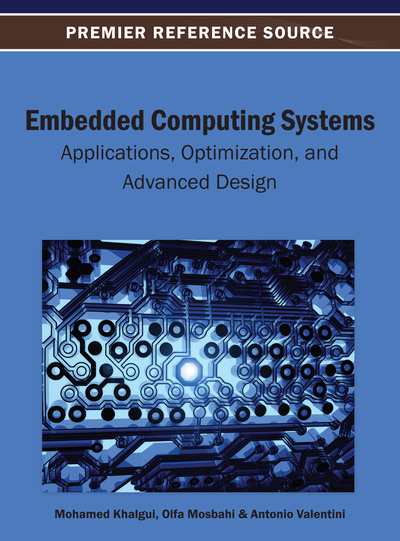# Expressing and Validating OCL Constraints using Graphs

Najet Zoubeir (Institut Supérieur d’Informatique, Tunisia), Adel Khalfallah (Institut Supérieur d’Informatique, Tunisia) and Samir Ben Ahmed (Tunis El Manar University, Tunisia)
DOI: 10.4018/978-1-4666-3922-5.ch005

## Abstract

The definition of the semantics of visual languages, in particular Unified Modeling Language (UML) diagrams, using graph formalism has known a wide success, since graphs fit the multi-dimensional nature of this kind of language. However, constraints written in Object Constraint Language (OCL) and defined on these models are still not well integrated within this graph-based semantics. In this chapter, the authors propose an integrated semantics of OCL constraints within class diagrams, using graph transformation systems. Their contribution is divided into two parts. In the first part, they introduce graph constraint patterns, as the translation into graphs of a subset of OCL expressions. These patterns are validated with experimental examples using the GROOVE toolset. In the second part, the authors define the relation between OCL and UML models within their graph transformation system.
Chapter Preview
Top

## 1. Introduction

The formal definition of the semantics of visual languages has been the focus of many works, in order to extend the scope of such languages to more critical domains. For example, UML diagrams have been formalized using different formalisms, such as formal specification languages (PVS [Aredo, 1999; Ledang & Souquires, 2001], CSP [Ng & Butler, 2003], Z [Dupuy, 2000; France & Bruel, 2001; France, Bruel, Larrondo-Petrie, & Grant, 1997],...). Among these formalisms, graph transformation systems have known a fair success representing the visual languages semantics, since this formalism is formal, universal and easily understood. Many graph-based semantics were proposed for UML diagrams, such as the Dynamic Meta-Modeling approach introduced by Hausmann, which formalizes many of the UML diagrams, such as the statechart diagrams (Engels, Hausmann, Heckel, & Sauer, 2000; Hausmann, 2001), the sequence diagrams (Hausmann, Heckel, & Sauer, 2002; Hausmann, Heckel, & Sauer, 2004) and the activity diagrams (Hausmann, 2005). Other works try rather to define integrated semantics for a number of UML diagrams. For example, (Kuske, Gogolla, Kollmann, & Kreowski, 2002), and (Gogolla, Ziemann, & Skuske, 2003) propose a graph-based integrated semantics for UML class, object and statechart diagrams, and (Holscher, Ziemann, & Gogolla, 2006) works on a larger subset of UML diagrams, including further the use cases and interaction diagrams.

## Complete Chapter List

Search this Book:
Reset